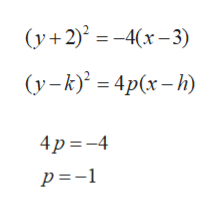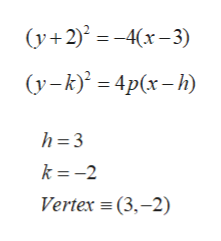# For Exercise, an equation of a parabola is given in standard form.a. Determine the value of pb. Identify the vertex.c. Identify the focus.d. Determine the focal diameter.e. Determine the endpoints of the latus rectum.f. Write an equation of the directrix.g. Write an equation for the axis of symmetry.h. Graph the parabola. (y + 2)2 = −4(x − 3).

Question
4 views

For Exercise, an equation of a parabola is given in standard form.

a. Determine the value of p

b. Identify the vertex.

c. Identify the focus.

d. Determine the focal diameter.

e. Determine the endpoints of the latus rectum.

f. Write an equation of the directrix.

g. Write an equation for the axis of symmetry.

h. Graph the parabola. (y + 2)2 = −4(x − 3).

check_circle

Step 1

Given equation of parabola in standard form:

Step 2

a.  Determine of the value of p.

Here is the standard form of parabola, where

(h,k) is vertex .

Now compare the given parabola equation with standard equation of parabola.

So, value of p = -1help_outlineImage Transcriptionclose(у+2)? %3- 4(х - 3) (у-к) — 4p(x- - h) 4 p =-4 p=-1 fullscreen
Step 3

b.  Identify the vertex.

Here (h,k) is vertex ...help_outlineImage Transcriptionclose(y+2) = -4(x–3) (y-k)' = 4p(x- h) h= 3 k = -2 Vertex = (3,–2) fullscreen

### Want to see the full answer?

See Solution

#### Want to see this answer and more?

Solutions are written by subject experts who are available 24/7. Questions are typically answered within 1 hour.*

See Solution
*Response times may vary by subject and question.
Tagged in

### Algebra